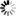Welcome to Central Library, SUST
Normal view

# Stochastic Calculus with Infinitesimals [electronic resource] / by Frederik Herzberg.

Material type:TextSeries: Lecture Notes in Mathematics ; 2067Publisher: Berlin, Heidelberg : Springer Berlin Heidelberg : Imprint: Springer, 2013Description: XVIII, 112 p. online resourceContent type:
• text
Media type:
• computer
Carrier type:
• online resource
ISBN:
• 9783642331497
Additional physical formats: Printed edition:: No titleDDC classification:
• 511.3 23
LOC classification:
• QA8.9-10.3
Online resources:
Contents:
1 Infinitesimal calculus, consistently and accessibly -- 2 Radically elementary probability theory -- 3 Radically elementary stochastic integrals -- 4 The radically elementary Girsanov theorem and the diffusion invariance principle -- 5 Excursion to nancial economics: A radically elementary approach to the fundamental theorems of asset pricing -- 6 Excursion to financial engineering: Volatility invariance in the Black-Scholes model -- 7 A radically elementary theory of Itô diffusions and associated partial differential equations -- 8 Excursion to mathematical physics: A radically elementary definition of Feynman path integrals -- 9 A radically elementary theory of Lévy processes -- 10 Final remarks.
Summary: Stochastic analysis is not only a thriving area of pure mathematics with intriguing connections to partial differential equations and differential geometry. It also has numerous applications in the natural and social sciences (for instance in financial mathematics or theoretical quantum mechanics) and therefore appears in physics and economics curricula as well. However, existing approaches to stochastic analysis either presuppose various concepts from measure theory and functional analysis or lack full mathematical rigour. This short book proposes to solve the dilemma: By adopting E. Nelson's "radically elementary" theory of continuous-time stochastic processes, it is based on a demonstrably consistent use of infinitesimals and thus permits a radically simplified, yet perfectly rigorous approach to stochastic calculus and its fascinating applications, some of which (notably the Black-Scholes theory of option pricing and the Feynman path integral) are also discussed in the book.
Tags from this library: No tags from this library for this title.
Star ratingsAverage rating: 0.0 (0 votes)
No physical items for this record

1 Infinitesimal calculus, consistently and accessibly -- 2 Radically elementary probability theory -- 3 Radically elementary stochastic integrals -- 4 The radically elementary Girsanov theorem and the diffusion invariance principle -- 5 Excursion to nancial economics: A radically elementary approach to the fundamental theorems of asset pricing -- 6 Excursion to financial engineering: Volatility invariance in the Black-Scholes model -- 7 A radically elementary theory of Itô diffusions and associated partial differential equations -- 8 Excursion to mathematical physics: A radically elementary definition of Feynman path integrals -- 9 A radically elementary theory of Lévy processes -- 10 Final remarks.

Stochastic analysis is not only a thriving area of pure mathematics with intriguing connections to partial differential equations and differential geometry. It also has numerous applications in the natural and social sciences (for instance in financial mathematics or theoretical quantum mechanics) and therefore appears in physics and economics curricula as well. However, existing approaches to stochastic analysis either presuppose various concepts from measure theory and functional analysis or lack full mathematical rigour. This short book proposes to solve the dilemma: By adopting E. Nelson's "radically elementary" theory of continuous-time stochastic processes, it is based on a demonstrably consistent use of infinitesimals and thus permits a radically simplified, yet perfectly rigorous approach to stochastic calculus and its fascinating applications, some of which (notably the Black-Scholes theory of option pricing and the Feynman path integral) are also discussed in the book.

There are no comments on this title.